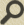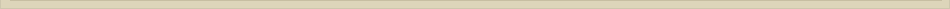Fundamentals of Advanced Mathematics

Introduction:

This course is designed to act as an introduction to proof based high-level mathematics. It guides students to think mathematically by analyzing a problem, extracting the pertinent information, and drawing appropriate conclusions.

This course will follow “A Transition to Advanced Mathematics” (Smith, Eggen, St. Andre; 6th Edition).

“In summary, our main goals in this text are to improve the student’s ability to think and write in a mature mathematical fashion and to provide a solid understanding of the material most useful for advanced coursed… in almost any mathematically related work you may do, the kind of reasoning you need to be able to do is the same reasoning you sue in proving theorems.” (Smith, preface)

I believe that understanding mathematics is a prerequisite to all other understanding.
My goal in this course is to convince you of the validity of this statement.

Content:Lesson 0: Introduction

Inductive vs Deductive reasoning AxiomsLesson 1: Propositions

Proposition Conjunction, disjunction, negationLesson 2: Truth Tables and Equivalence

Propositional forms Well-formed Truth tables Equivalence Tautology and ContradictionProblem Set 1Lesson 3: Conditionals and Biconditionals

Conditional and biconditional ConverseLesson 4: Contrapositive and Equivalence

Modus Ponens and Modus Tollens Contrapositive Equivilance Theorem 0.0 Theorem 1.1Problem Set 2

Modus Ponens and Modus TollensLesson 5: Open Sentences and QuantifiersLesson 6: Denials and Equivalence of Quantifiers